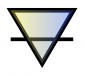# Automatic rotation of moving objects to match their direction

Hi there. I'm a noob to 3DS Max, and have hit a wall with this. I'm using external position data (csv) to animate objects in 3D space. I've managed to get objects moving ok, but I cannot figure out how to rotate them to face in the direction of travel. I've seen some other problem pages about this, but couldn't get their scripts working.

Very grateful for any assistance. I include my code below for an idea of where I stand.

Robin

------------------

resetMaxFile #noPrompt
(
local theFile = openFile "p:\\data.csv"
global data = #()
global points = #()
while not eof theFile do (
append data t
append points p
)
close theFile
)

(
--Setup objects
for i = 1 to 50 do
(
Box lengthsegs:1 widthsegs:1 heightsegs:1 length:5 width:5 height:5 mapcoords:on pos:[points[i], points[i], points[i]] isSelected:on
\$Box001.name = ((i as integer) as string)
)
)

--Animate objects
animate on
(
k = 1
for t in 1 to 100 do
(
at time (t)
(
for i in 1 to 50 do
(
execute ("select \$" + (data[k] as integer) as string)
\$.pos = points[k]
k = k+1
if k > 5000 do
(
k = 1
)
)
)
)
)

## Comment viewing options### object orientation

actually is more easy to orient on object and this method will work on all axis:

resetMaxFile #noPrompt

(
--Setup object
Box lengthsegs:1 widthsegs:1 heightsegs:1 length:10 width:30 height:10 mapcoords:on pos:[50, 0, 0] isSelected:on
)

--Animate objects
animate on
(
animationRange = interval 1 360
for t in 1 to 360 do
(
at time (t)
(
\$Box001.pos = [cos(t)*50, sin(t)*80, sin(4*t)*20]
now = \$Box001.pos

if(t > 1) then
(
dirVec = normalize (now - last)

\$Box001.dir = dirVec

)
global last = now
)
)
)### Problem solved..

..by (a) calculating object's current direction from the current and last-frame positions, and (b) rotating using script from Bobo*, as follows:

resetMaxFile #noPrompt

(
--Setup object
Box lengthsegs:1 widthsegs:1 heightsegs:1 length:10 width:30 height:10 mapcoords:on pos:[50, 0, 0] isSelected:on
)

--Animate objects
animate on
(
animationRange = interval 1 360
for t in 1 to 360 do
(
at time (t)
(
\$Box001.pos = [cos(t)*50, sin(t)*50, 0]
now = \$Box001.pos

if(t > 1) then
(
dirVec = normalize (now - last)
--ang = asin(dirVec/50)

dir = atan (dirVec.y/dirVec.x)
if dirVec.x < 0 and dirVec.y > 0 then dir = 180+dir
if dirVec.x < 0 and dirVec.y < 0 then dir = -180+dir

if(t>2) then
(
ang = dir-lastdir
rot_obj = eulerangles 0 0 ang
)
else rot_obj = eulerangles 0 0 dir
rotate \$Box001 rot_obj
)
global last = now
global lastdir = dir
)
)
)### With my method you are

With my method you are calculating direction from given data on the fly.
And i think is a faster solution then calculating for each object all over again same code. Maybe i'm wrong but check from youself.
By the way your code not working

barigazy### Any idea how I kill this thread?

There doesn't appear to be an option anywhere### Thanks barigazy

I mostly cracked this problem, but my new post has the updated version..### Here we go

```(
local dirArr = #(), objArr = #()
for i = 1 to 50 do
(
--b = Box lengthsegs:1 widthsegs:1 heightsegs:1 length:5 width:5 height:5 mapcoords:on isSelected:off name: (i as string)
b = Pyramid widthsegs:1 depthSegs:1 heightsegs:1 width:10 depth:10 height:10 isSelected:off name: (i as string)
append objArr b ; append dirArr [0,0,0]
)
local dataF = openFile "p:\\data.txt", curFrame = 0
while not eof dataF do
(
local obj = objArr[id]
with animate on (at time frames (obj.pos = pos ; obj.dir = dir))
deletekey obj.track 1
curFrame += frames ; dirArr[id] = pos
)
close f ; free dirArr ; free objArr
)```

barigazy### I don't have *.csv to do some

I don't have *.csv to do some test but hope this help

```resetMaxFile #noPrompt
(
local theFile = openFile "p:\\data.csv"
global data = #()
global points = #()
while not eof theFile do
(
append data t
append points p
)
close theFile
)

--Setup objects
for i = 1 to 50 do
(
Box lengthsegs:1 widthsegs:1 heightsegs:1 length:5 width:5 height:5 mapcoords:on \
pos:[points[i], points[i], points[i]] isSelected:on name: (i as string)
)

--Animate objects
animate on
(
k = 1
for t in 1 to 100 do
(
at time (t)
(
for i in 1 to 50 do
(
obj = getnodebyname (data[k] as integer)
obj.pos = points[k]
if k < 5000 do obj.dir = points[k+1]-points[k]
k +=1
if k > 5000 do k = 1
(

)
)
)```

barigazy### Thanks but not quite!

Thanks for your effort. Unfortunately that killed the animation completely!

I attach the data file so you can test the script (now a txt file so the script will need updating).

AttachmentSize
data.txt 181.3 KB### can you explain the numbers

can you explain the numbers inside *.txt (first and second in the line).
3rd,4th and 5th are position coords, right?

barigazy### Sure..

The 1st column is time (frame). I don't think the script uses it: there are exactly 50 objects repeating every frame, so I just used a regular loop instead. The 2nd column is object ID, which is used in the script to select the correct box to update its position.

Apologies if the script is inefficient or unconventional - it is my first maxscript!